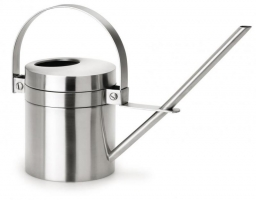# Watering 7078

A full watering can weigh 27kg. When half full, it weighs 15 kg. How much can an empty watering weigh?

k =  3 kg

### Step-by-step explanation:

k+v = 27
k + v/2 = 15

k+v = 27
2k+v = 30

Pivot: Row 1 ↔ Row 2
2k+v = 30
k+v = 27

Row 2 - 1/2 · Row 1 → Row 2
2k+v = 30
0.5v = 12

v = 12/0.5 = 24
k = 30-v/2 = 30-24/2 = 3

k = 3
v = 24

Our linear equations calculator calculates it.Did you find an error or inaccuracy? Feel free to write us. Thank you!

Tips for related online calculators
Do you have a linear equation or system of equations and looking for its solution? Or do you have a quadratic equation?